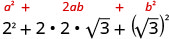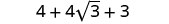# 9.4: Multiply Square Roots

•• OpenStax
• OpenStax
$$\newcommand{\vecs}{\overset { \rightharpoonup} {\mathbf{#1}} }$$ $$\newcommand{\vecd}{\overset{-\!-\!\rightharpoonup}{\vphantom{a}\smash {#1}}}$$$$\newcommand{\id}{\mathrm{id}}$$ $$\newcommand{\Span}{\mathrm{span}}$$ $$\newcommand{\kernel}{\mathrm{null}\,}$$ $$\newcommand{\range}{\mathrm{range}\,}$$ $$\newcommand{\RealPart}{\mathrm{Re}}$$ $$\newcommand{\ImaginaryPart}{\mathrm{Im}}$$ $$\newcommand{\Argument}{\mathrm{Arg}}$$ $$\newcommand{\norm}{\| #1 \|}$$ $$\newcommand{\inner}{\langle #1, #2 \rangle}$$ $$\newcommand{\Span}{\mathrm{span}}$$ $$\newcommand{\id}{\mathrm{id}}$$ $$\newcommand{\Span}{\mathrm{span}}$$ $$\newcommand{\kernel}{\mathrm{null}\,}$$ $$\newcommand{\range}{\mathrm{range}\,}$$ $$\newcommand{\RealPart}{\mathrm{Re}}$$ $$\newcommand{\ImaginaryPart}{\mathrm{Im}}$$ $$\newcommand{\Argument}{\mathrm{Arg}}$$ $$\newcommand{\norm}{\| #1 \|}$$ $$\newcommand{\inner}{\langle #1, #2 \rangle}$$ $$\newcommand{\Span}{\mathrm{span}}$$$$\newcommand{\AA}{\unicode[.8,0]{x212B}}$$

##### Learning Objectives

By the end of this section, you will be able to:

• Multiply square roots
• Use polynomial multiplication to multiply square roots
##### Note

Before you get started, take this readiness quiz.

1. Simplify: (3u)(8v).
If you missed this problem, review Example 6.2.31.
2. Simplify: 6(12−7n).
If you missed this problem, review Example 6.3.1.
3. Simplify: (2+a)(4−a).
If you missed this problem, review Example 6.3.34.

## Multiply Square Roots

We have used the Product Property of Square Roots to simplify square roots by removing the perfect square factors. The Product Property of Square Roots says

$\sqrt{ab}=\sqrt{a}·\sqrt{b} \nonumber$

We can use the Product Property of Square Roots ‘in reverse’ to multiply square roots.

$\sqrt{a}·\sqrt{b}=\sqrt{ab} \nonumber$

Remember, we assume all variables are greater than or equal to zero.

We will rewrite the Product Property of Square Roots so we see both ways together.

##### Definition: PRODUCT PROPERTY OF SQUARE ROOTS

If a, b are nonnegative real numbers, then

$$\sqrt{ab}=\sqrt{a}·\sqrt{b}$$ and $$\sqrt{a}·\sqrt{b}=\sqrt{ab}$$.

So we can multiply $$\sqrt{3}·\sqrt{5}$$ in this way:

$\begin{array}{l} {\sqrt{3}·\sqrt{5}}\\ {\sqrt{3·5}}\\ {\sqrt{15}}\\ \nonumber \end{array}$

Sometimes the product gives us a perfect square:

$\begin{array}{l} {\sqrt{2}·\sqrt{8}}\\ {\sqrt{2·8}}\\ {\sqrt{16}}\\ {4}\\ \nonumber \end{array}$

Even when the product is not a perfect square, we must look for perfect-square factors and simplify the radical whenever possible.

Multiplying radicals with coefficients is much like multiplying variables with coefficients. To multiply 4x·3y we multiply the coefficients together and then the variables. The result is 12xy. Keep this in mind as you do these examples.

##### Example $$\PageIndex{1}$$

Simplify:

1. $$\sqrt{2}·\sqrt{6}$$
2. $$(4\sqrt{3})(2\sqrt{12})$$.
 1. $$\sqrt{2}·\sqrt{6}$$ Multiply using the Product Property. $$\sqrt{12}$$ Simplify the radical. $$\sqrt{4}·\sqrt{3}$$ Simplify. $$2\sqrt{3}$$ 2. $$(4\sqrt{3})(2\sqrt{12})$$ Multiply using the Product Property. $$8\sqrt{36}$$ Simplify the radical. $$8·6$$ Simplify. $$48$$
##### Example $$\PageIndex{2}$$

Simplify:

1. $$\sqrt{3}·\sqrt{6}$$
2. $$(2\sqrt{6})(3\sqrt{12})$$.
1. $$3\sqrt{2}$$
2. $$36\sqrt{2}$$
##### Example $$\PageIndex{3}$$

Simplify:

1. $$\sqrt{5}·\sqrt{10}$$
2. $$(6\sqrt{3})(5\sqrt{6})$$
1. $$5\sqrt{2}$$
2. $$90\sqrt{2}$$
##### Example $$\PageIndex{4}$$

Simplify: $$(6\sqrt{2})(3\sqrt{10})$$

 $$(6\sqrt{2})(3\sqrt{10})$$ Multiply using the Product Property. $$18\sqrt{20}$$ Simplify the radical. $$18\sqrt{4}·\sqrt{5}$$ Simplify. $$18·2·\sqrt{5}$$ $$36\sqrt{5}$$
##### Example $$\PageIndex{5}$$

Simplify: $$(3\sqrt{2})(2\sqrt{30})$$

$$12\sqrt{15}$$

##### Example $$\PageIndex{6}$$

Simplify: $$(3\sqrt{3})(3\sqrt{6})$$.

$$27\sqrt{2}$$

When we have to multiply square roots, we first find the product and then remove any perfect square factors.

##### Example $$\PageIndex{7}$$

Simplify:

1. $$(\sqrt{8x^3})(\sqrt{3x})$$
2. $$(\sqrt{20y^2})(\sqrt{5y^3})$$
 1. $$(\sqrt{8x^3})(\sqrt{3x})$$ Multiply using the Product Property. $$\sqrt{24x^4}$$ Simplify the radical. $$\sqrt{4x^4}·\sqrt{6}$$ Simplify. $$2x^2\sqrt{6}$$ 2. $$(\sqrt{20y^2})(\sqrt{5y^3})$$ Multiply using the Product Property. $$\sqrt{100y^5}$$ Simplify the radical. $$10y^2\sqrt{y}$$
##### Example $$\PageIndex{8}$$

Simplify:

1. $$(\sqrt{6x^3})(\sqrt{3x})$$
2. $$(\sqrt{2y^3})(\sqrt{50y^2})$$.
1. $$3x^2\sqrt{2}$$
2. $$10y^2\sqrt{y}$$
##### Example $$\PageIndex{9}$$

Simplify:

1. $$(\sqrt{6x^5})(\sqrt{2x})$$
2. $$(\sqrt{12y^2})(\sqrt{3y^5})$$
1. $$2x^3\sqrt{3}$$
2. $$6y^2\sqrt{y}$$
##### Example $$\PageIndex{10}$$

Simplify: $$(10\sqrt{6p^3})(3\sqrt{18p})$$

 $$(10\sqrt{6p^3})(3\sqrt{18p})$$ Multiply. $$30\sqrt{108p^4}$$ Simplify the radical. $$30\sqrt{36p^4}·\sqrt{3}$$ $$30·6p^2·\sqrt{3}$$ $$180p^2\sqrt{3}$$
##### Example $$\PageIndex{11}$$

Simplify: $$(\sqrt{62x^2})(8\sqrt{45x^4})$$

$$144x^3\sqrt{10}$$

##### Example $$\PageIndex{12}$$

Simplify: $$(2\sqrt{6y^4})(12\sqrt{30y})$$.

$$144y^2\sqrt{5y}$$

##### Example $$\PageIndex{13}$$

Simplify:

1. $$(\sqrt{2})^2$$
2. $$(−\sqrt{11})^2$$.
 1. $$(\sqrt{2})^2$$ Rewrite as a product. $$(\sqrt{2})(\sqrt{2})$$ Multiply. $$\sqrt{4}$$ Simplify. 2 2. $$(−\sqrt{11})^2$$ Rewrite as a product. $$(−\sqrt{11})(−\sqrt{11})$$ Multiply. $$\sqrt{121}$$ Simplify. 11
##### Example $$\PageIndex{14}$$

Simplify:

1. $$(\sqrt{12})^2$$
2. $$(−\sqrt{15})^2$$.
1. 12
2. 15
##### Example $$\PageIndex{15}$$

Simplify:

1. $$(\sqrt{16})^2$$
2. $$(−\sqrt{20})^2$$.
1. 16
2. 20

The results of the previous example lead us to this property.

##### Definition: SQUARING A SQUARE ROOT

If a is a nonnegative real number, then

$$(\sqrt{a})^2=a$$

By realizing that squaring and taking a square root are ‘opposite’ operations, we can simplify $$(\sqrt{2})^2$$ and get 2 right away. When we multiply the two like square roots in part (a) of the next example, it is the same as squaring.

##### Example $$\PageIndex{16}$$

Simplify:

1. $$(2\sqrt{3})(8\sqrt{3})$$
2. $$(3\sqrt{6})^2$$.
 1. $$(2\sqrt{3})(8\sqrt{3})$$ Multiply. Remember, $$(\sqrt{3}^2)$$ 16·3 Simplify. 48 2. $$(3\sqrt{6})^2$$ Multiply. 9·6 Simplify. 54
##### Example $$\PageIndex{17}$$

Simplify:

1. $$(6\sqrt{11})(5\sqrt{11})$$
2. $$(5\sqrt{8})^2$$.
1. 330
2. 200
##### Example $$\PageIndex{18}$$

Simplify:

1. $$(3\sqrt{7})(10\sqrt{7})$$
2. $$(−4\sqrt{6})^2$$.
1. 210
2. 96

## Use Polynomial Multiplication to Multiply Square Roots

In the next few examples, we will use the Distributive Property to multiply expressions with square roots.

We will first distribute and then simplify the square roots when possible.

##### Example $$\PageIndex{19}$$

Simplify:

1. $$3(5−\sqrt{2})$$
2. $$\sqrt{2}(4−\sqrt{10})$$.
 1. $$3(5−\sqrt{2})$$ Distribute. $$15−3\sqrt{2})$$ 2. $$\sqrt{2}(4−\sqrt{10})$$ Distribute. $$4\sqrt{2}−\sqrt{20}$$ Simplify. $$4\sqrt{2}−2\sqrt{5}$$
##### Example $$\PageIndex{20}$$

Simplify:

1. $$2(3−\sqrt{5})$$
2. $$\sqrt{3}(2−\sqrt{18})$$.
1. $$6−2\sqrt{5}$$
2. $$2\sqrt{3}−3\sqrt{6}$$
##### Example $$\PageIndex{21}$$

Simplify:

1. $$6(2+\sqrt{6})$$
2. $$\sqrt{7}(1+\sqrt{14})$$.
1. $$12+\sqrt{6}$$
2. $$\sqrt{7}+7\sqrt{2}$$
##### Example $$\PageIndex{22}$$

Simplify:

1. $$\sqrt{5}(7+2\sqrt{5})$$
2. $$\sqrt{6}(\sqrt{2}+\sqrt{18})$$.
 1. $$\sqrt{5}(7+2\sqrt{5})$$ Distribute. $$7\sqrt{5}+2·5$$ Simplify. $$7\sqrt{5}+10$$ $$10+7\sqrt{5}$$ 2. $$\sqrt{6}(\sqrt{2}+\sqrt{18})$$ Multiply. $$\sqrt{12}+\sqrt{108}$$ Simplify. $$(\sqrt{4}·\sqrt{3}+\sqrt{36}·\sqrt{3})$$ $$2\sqrt{3}+6\sqrt{3}$$ Combine like radicals. $$8\sqrt{3}$$
##### Example $$\PageIndex{23}$$

Simplify:

1. $$\sqrt{6}(1+3\sqrt{6})$$
2. $$\sqrt{12}(\sqrt{3}+\sqrt{24})$$
1. $$18+\sqrt{6}$$
2. $$6+12\sqrt{2}$$
##### Example $$\PageIndex{24}$$

Simplify:

1. $$\sqrt{8}(2−5\sqrt{8})$$
2. $$\sqrt{14}(\sqrt{2}+\sqrt{42})$$
1. $$−40+4\sqrt{2}$$
2. $$2\sqrt{7}+14\sqrt{3}$$

When we worked with polynomials, we multiplied binomials by binomials. Remember, this gave us four products before we combined any like terms. To be sure to get all four products, we organized our work—usually by the FOIL method.

##### Example $$\PageIndex{25}$$

Simplify: $$(2+\sqrt{3})(4−\sqrt{3})$$

 $$(2+\sqrt{3})(4−\sqrt{3})$$ Multiply. $$8−2\sqrt{3}+4\sqrt{3}−3$$ Combine like terms. $$5+2\sqrt{3}$$
##### Example $$\PageIndex{26}$$

Simplify: $$(1+\sqrt{6})(3−\sqrt{6})$$.

$$−3+2\sqrt{6}$$

##### Example $$\PageIndex{27}$$

Simplify: $$(4−\sqrt{10})(2+\sqrt{10})$$.

$$−2+2\sqrt{10}$$

##### Example $$\PageIndex{28}$$

Simplify: $$(3−2\sqrt{7})(4−2\sqrt{7})$$.

 $$(3−2\sqrt{7})(4−2\sqrt{7})$$ Multiply. $$12−6\sqrt{7}−8\sqrt{7}+4·7$$ Simplify. $$12−6\sqrt{7}−8\sqrt{7}+28$$ Combine like terms. $$40−14\sqrt{7}$$
##### Example $$\PageIndex{29}$$

Simplify: $$(6−3\sqrt{7})(3+4\sqrt{7})$$.

$$−66+15\sqrt{7}$$

##### Example $$\PageIndex{30}$$

Simplify: $$(2−3\sqrt{11})(4−\sqrt{11})$$

$$41+14\sqrt{11}$$

##### Example $$\PageIndex{31}$$

Simplify: $$(3\sqrt{2}−\sqrt{5})(\sqrt{2}+4\sqrt{5})$$.

 $$3\sqrt{2}−\sqrt{5})(\sqrt{2}+4\sqrt{5})$$ Multiply. $$3·2+12\sqrt{10}−\sqrt{10}−4·5$$ Simplify. $$6+12\sqrt{10}−\sqrt{10}−20$$ Combine like terms. $$−14+11\sqrt{10}$$
##### Example $$\PageIndex{32}$$

Simplify: $$(5\sqrt{3}−\sqrt{7})(\sqrt{3}+2\sqrt{7})$$

$$1+9\sqrt{21}$$

##### Example $$\PageIndex{33}$$

Simplify: $$(\sqrt{6}−3\sqrt{8})(2\sqrt{6}+\sqrt{8})$$

$$−12−20\sqrt{3}$$

##### Example $$\PageIndex{34}$$

Simplify: $$(4−2\sqrt{x})(1+3\sqrt{x})$$.

 $$(4−2\sqrt{x})(1+3\sqrt{x})$$. Multiply. $$4+12\sqrt{x}−2\sqrt{x}−6x$$ Combine like terms. $$4+10\sqrt{x}−6x$$
##### Example $$\PageIndex{35}$$

Simplify: $$(6−5\sqrt{m})(2+3\sqrt{m})$$.

$$12+8\sqrt{m}−15m$$

##### Example $$\PageIndex{36}$$

Simplify: $$(10+3\sqrt{n})(1−5\sqrt{n})$$

$$10−47\sqrt{n}−15n$$

Note that some special products made our work easier when we multiplied binomials earlier. This is true when we multiply square roots, too. The special product formulas we used are shown below.

##### Definition: SPECIAL PRODUCT FORMULAS

$\begin{array}{ll} {\textbf{Binomial Squares}}&{\textbf{Product of Conjugates}}\\ {(a+b)^2=a^2+2ab+b^2}&{(a−b)(a+b)=a^2−b^2}\\ {(a−b)^2=a^2−2ab+b^2}&{}\\ \nonumber \end{array}$

We will use the special product formulas in the next few examples. We will start with the Binomial Squares formula.

##### Example $$\PageIndex{37}$$

Simplify:

1. $$(2+\sqrt{3})^2$$
2. $$(4−2\sqrt{5})^2$$.

Be sure to include the 2ab term when squaring a binomial.

1.Multiply using the binomial square pattern.Simplify.Combine like terms.2.Multiply using the binomial square pattern.Simplify.Combine like terms.##### Example $$\PageIndex{38}$$

Simplify:

1. $$(10+\sqrt{2})^2$$
2. $$(1+3\sqrt{6})^2$$.
1. $$102+20\sqrt{2}$$
2. $$55+6\sqrt{6}$$
##### Exercise $$\PageIndex{39}$$

Simplify:

1. $$(6-\sqrt{5})^2$$
2. $$(9-2\sqrt{10})^2$$.
1. $$41-12\sqrt{5}$$
2. $$121-36\sqrt{10}$$
##### Example $$\PageIndex{40}$$

Simplify: $$(1+3\sqrt{x})^2$$.Multiply using the binomial square pattern.Simplify.##### Example $$\PageIndex{41}$$

Simplify: $$(2+5\sqrt{m})^2$$.

$$4+20\sqrt{m}+25m$$

##### Example $$\PageIndex{42}$$

Simplify: $$(3−4\sqrt{n})^2$$.

$$9−24\sqrt{n}+16n$$

In the next two examples, we will find the product of conjugates.

##### Example $$\PageIndex{43}$$

Simplify: $$(4−\sqrt{2})(4+\sqrt{2})$$.Multiply using the binomial square pattern.Simplify.##### Example $$\PageIndex{44}$$

Simplify: $$(2−\sqrt{3})(2+\sqrt{3})$$

1

##### ExAMPLe $$\PageIndex{45}$$

Simplify: $$(1+\sqrt{5})(1−\sqrt{5})$$

−4

##### Example $$\PageIndex{46}$$

Simplify: $$(5−2\sqrt{3})(5+2\sqrt{3})$$Multiply using the binomial square pattern.Simplify.##### Example $$\PageIndex{47}$$

Simplify: $$(3−2\sqrt{5})(3+2\sqrt{5})$$.

−11

##### Example $$\PageIndex{48}$$

Simplify: $$(4+5\sqrt{7})(4−5\sqrt{7})$$.

−159

Access these online resources for additional instruction and practice with multiplying square roots.

• Product Property
• Multiply Binomials with Square Roots

## Key Concepts

• Product Property of Square Roots If a, b are nonnegative real numbers, then

$$\sqrt{ab}=\sqrt{a·b}$$ and $$\sqrt{a}·\sqrt{b}=\sqrt{ab}$$

• Special formulas for multiplying binomials and conjugates:

$\begin{array}{ll} {(a+b)^2=a^2+2ab+b^2}&{(a−b)(a+b)=a^2−b^2}\\ {(a−b)^2=a^2−2ab+b^2 }&{}\\ \nonumber \end{array}$

• The FOIL method can be used to multiply binomials containing radicals.

This page titled 9.4: Multiply Square Roots is shared under a CC BY-NC-SA license and was authored, remixed, and/or curated by OpenStax.